### Problem Statement

Hieroglyphs were widely used for writing in ancient times and they are used in some languages even now. In this problem, we will consider a simplified model of hieroglyphs.

Let's call a hieroglyph a two-dimensional figure that consists of several segments, each of which has a positive length and is either horizontal or vertical. The segments in the same hieroglyph can touch or intersect each other, but no two segments may overlap. That is, there are no two segments such that the length of their intersection is positive. The segments are considered to be infinitely thin.

You will be given two hieroglyphs in String[] hier1 and hier2. Both of them are drawn on the same plane with a Cartesian coordinate system. Each element of hier1 will be a comma-separated list of segments. Each segment is formatted "x1 y1 x2 y2" (quotes for clarity), where (x1, y1) and (x2, y2) are the coordinates of its two endpoints (x1 ≤ x2, y1 ≤ y2). The set of all segments of the first hieroglyph is the union of all segments presented in elements of hier1. The set of all segments of the second hieroglyph is given in the same format in elements of hier2. It is guaranteed that both hier1 and hier2 describe valid hieroglyphs as defined in the previous paragraph.

You are allowed to move each of the hieroglyphs to an arbitrary place on the plane via translation (See notes). No other operations like applying rotation or symmetry are allowed. Once the positions for each hieroglyph are chosen, their union is defined as a set of points on the plane that belong to at least one of them. It's easy to see that union of two hieroglyphs can be represented as a set of non-overlapping segments. You are to minimize the total length of these segments.

Return this minimum possible total length.

### Definition

 Class: Hieroglyphs Method: minimumVisible Parameters: String[], String[] Returns: int Method signature: int minimumVisible(String[] hier1, String[] hier2) (be sure your method is public)

### Notes

-Translation is an operation that moves every point a constant distance in a specified direction. More exactly, an arbitrary vector (dx, dy) is first chosen, and then translation works as moving each point (x, y) to (x + dx, y + dy).

### Constraints

-hier1 and hier2 will each contain between 1 and 50 elements, inclusive.
-Each element of hier1 and hier2 will contain between 1 and 50 characters, inclusive.
-Each element of hier1 and hier2 will be a single comma separated list of between 1 and 4 segment descriptions, inclusive, without leading and trailing commas.
-Each segment description will be formatted "x1 y1 x2 y2" (quotes for clarity), where x1, y1, x2 and y2 are integers between 0 and 80, inclusive, with no extra leading zeros.
-In each segment description, x1 will be less than or equal to x2 and y1 will be less than or equal to y2.
-Each segment will be either horizontal or vertical and will have a positive length.
-No two segments of the same hieroglyph will overlap (as defined in the statement).

### Examples

0)

 `{"0 0 10 0,10 0 10 3"}` `{"0 1 10 1","5 1 5 4"}`
`Returns: 16`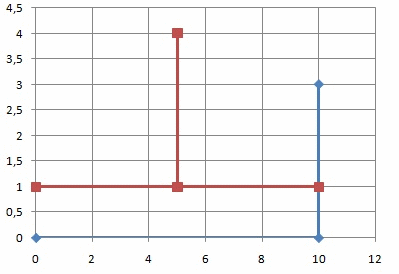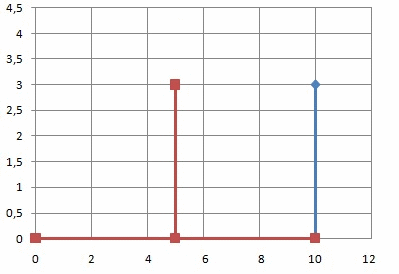Here it's better to combine the horizontal line segments than the vertical ones.
1)

 `{"1 1 1 5"}` `{"3 2 5 2"}`
`Returns: 6`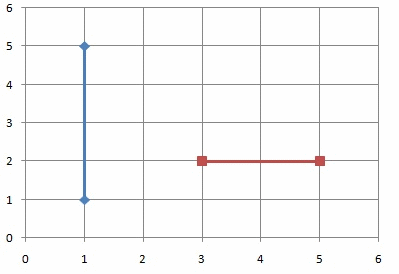There is no way to combine the hieroglyphs in such a way that segments overlap.
2)

 `{"0 2 6 2"}` `{"5 1 6 1,8 1 9 1"}`
`Returns: 6`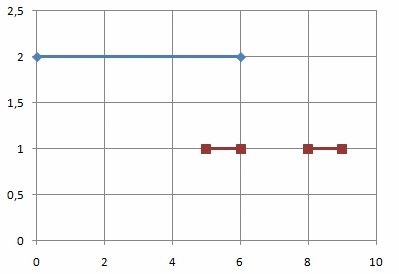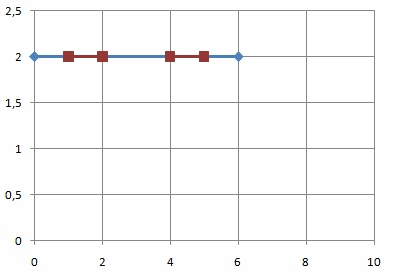3)

 `{"10 20 10 30,15 20 15 30","10 20 15 20,0 30 30 30"}` ```{"0 5 0 15,5 5 5 25","0 5 5 5,0 15 5 15"} ```
`Returns: 65`
4)

 `{"10 10 10 20,10 30 10 40","10 10 20 10"}` `{"10 0 10 20,10 27 10 35","10 0 20 0"}`
`Returns: 45`

#### Problem url:

http://www.topcoder.com/stat?c=problem_statement&pm=11230

#### Problem stats url:

http://www.topcoder.com/tc?module=ProblemDetail&rd=14243&pm=11230

Chmel_Tolstiy

#### Testers:

PabloGilberto , ivan_metelsky , pieguy

#### Problem categories:

Simple Search, Iteration